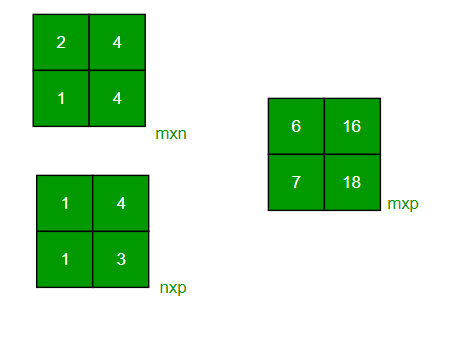# C Program to multiply two matrices

• Last Updated : 26 Dec, 2021

Given two matrices, the task to multiply them. Matrices can either be square or rectangular.

Examples:

```Input : mat1[][] = {{1, 2},
{3, 4}}
mat2[][] = {{1, 1},
{1, 1}}
Output : {{3, 3},
{7, 7}}
Input : mat1[][] = {{2, 4},
{3, 4}}
mat2[][] = {{1, 2},
{1, 3}}
Output : {{6, 16},
{7, 18}}```Multiplication of Square Matrices :
The below program multiplies two square matrices of size 4*4, we can change N for different dimensions.

## C

 `// C program to multiply two square matrices.``#include ``#define N 4`` ` `// This function multiplies mat1[][] and mat2[][],``// and stores the result in res[][]``void` `multiply(``int` `mat1[][N], ``int` `mat2[][N], ``int` `res[][N])``{``    ``int` `i, j, k;``    ``for` `(i = 0; i < N; i++) {``        ``for` `(j = 0; j < N; j++) {``            ``res[i][j] = 0;``            ``for` `(k = 0; k < N; k++)``                ``res[i][j] += mat1[i][k] * mat2[k][j];``        ``}``    ``}``}`` ` `int` `main()``{``    ``int` `mat1[N][N] = { { 1, 1, 1, 1 },``                       ``{ 2, 2, 2, 2 },``                       ``{ 3, 3, 3, 3 },``                       ``{ 4, 4, 4, 4 } };`` ` `    ``int` `mat2[N][N] = { { 1, 1, 1, 1 },``                       ``{ 2, 2, 2, 2 },``                       ``{ 3, 3, 3, 3 },``                       ``{ 4, 4, 4, 4 } };`` ` `    ``int` `res[N][N]; ``// To store result``    ``int` `i, j;``    ``multiply(mat1, mat2, res);`` ` `    ``printf``("Result matrix is ``");``    ``for` `(i = 0; i < N; i++) {``        ``for` `(j = 0; j < N; j++)``            ``printf``(``"%d "``, res[i][j]);``        ``printf``("``");``    ``}`` ` `    ``return` `0;``}`
Output
```Result matrix is
10 10 10 10
20 20 20 20
30 30 30 30
40 40 40 40```

Time complexity: O(n3). It can be optimized using Strassen’s Matrix Multiplication

Auxiliary Space: O(n2)

Multiplication of Rectangular Matrices :
We use pointers in C to multiply to matrices. Please refer to the following post as a prerequisite of the code.
How to pass a 2D array as a parameter in C?

## C

 `// C program to multiply two rectangular matrices``#include `` ` `// Multiplies two matrices mat1[][] and mat2[][]``// and prints result.``// (m1) x (m2) and (n1) x (n2) are dimensions``// of given matrices.``void` `multiply(``int` `m1, ``int` `m2, ``int` `mat1[][m2], ``int` `n1,``              ``int` `n2, ``int` `mat2[][n2])``{``    ``int` `x, i, j;``    ``int` `res[m1][n2];``    ``for` `(i = 0; i < m1; i++) ``    ``{``        ``for` `(j = 0; j < n2; j++) ``        ``{``            ``res[i][j] = 0;``            ``for` `(x = 0; x < m2; x++) ``            ``{``                ``*(*(res + i) + j) += *(*(mat1 + i) + x)``                                     ``* *(*(mat2 + x) + j);``            ``}``        ``}``    ``}``    ``for` `(i = 0; i < m1; i++) ``    ``{``        ``for` `(j = 0; j < n2; j++) ``        ``{``            ``printf``(``"%d "``, *(*(res + i) + j));``        ``}``        ``printf``("``");``    ``}``}`` ` `// Driver code``int` `main()``{``    ``int` `mat1[] = { { 2, 4 }, { 3, 4 } };``    ``int` `mat2[] = { { 1, 2 }, { 1, 3 } };``    ``int` `m1 = 2, m2 = 2, n1 = 2, n2 = 2;``   ` `    ``// Function call``    ``multiply(m1, m2, mat1, n1, n2, mat2);``    ``return` `0;``}`
Output
```6 16
7 18```

Time complexity: O(n3). It can be optimized using Strassen’s Matrix Multiplication

Auxiliary Space: O(m1 * n2)

Please refer complete article on Program to multiply two matrices for more details!

My Personal Notes arrow_drop_up